# Texas Go Math Grade 4 Unit 3 Answer Key Algebraic Reasoning

Refer to our Texas Go Math Grade 4 Answer Key Pdf to score good marks in the exams. Test yourself by practicing the problems from Texas Go Math Grade 4 Unit 3 Answer Key Algebraic Reasoning.

## Texas Go Math Grade 4 Unit 3 Answer Key Algebraic Reasoning

Show What You Know

Missing Factors Find the missing factor.

Question 1.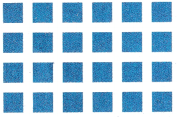___________ × 6 = 24
4× 6 = 24
Explanation:
4 rows and 6 columns
Each row consists of 6 tiles, total 4 x 6 = 24 tiles

Question 2.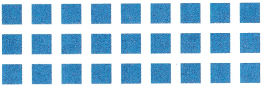3 × ___________ = 27
3 × 9 = 27
Explanation:
3 rows and 9 columns
Each row consists of 9 tiles, total 3 x 9 = 27 tiles

Find Perimeter Add to find the perimeter.

Question 3.Perimeter = ____________
12 units
Explanation:
Perimeter = 2(length + width)
= 2 (2 + 4 )
= 2(6)
= 12

Question 4.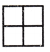Perimeter = ____________
8 units
Explanation:
Perimeter = 2(length + width)
= 2 (2 + 2 )
= 2(4)
= 8

Question 5.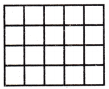Perimeter = ____________
16 units
Explanation:
Perimeter = 2(length + width)
= 2 (4 + 4 )
= 2(8)
= 16

Question 6.Perimeter = ____________
16 units
Explanation:
Perimeter = 2(length + width)
= 2 (2 + 6 )
= 2(8)
= 16

Find Area of Rectangles Find the area.

Question 7.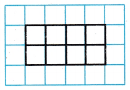Area = ____________ square units
8 square units
Explanation:
Area = (Length x Width)  square units
= 2 x 4
= 8 square units

Question 8.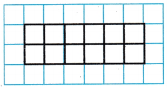Area = ____________ square units
12 square units
Explanation:
Area = (Length x Width)  square units
= 2 x 6
= 12 square units

Vocabulary Builder

Review Words

• unit
Measurement is to find a number that shows the amount of something. A measurement unit is a standard quantity used to express a physical quantity.

Preview Words

• area
• formula
• length
• pattern
• perimeter
• square unit
• term
• width

Visualize It

Place the checked words in the appropriate columns of the table. Words may be used more than once.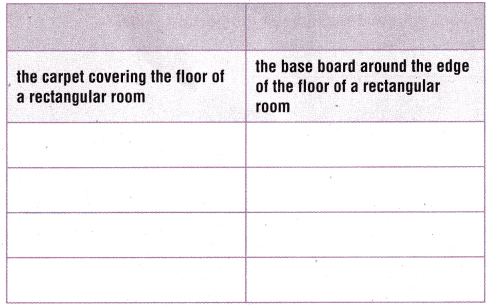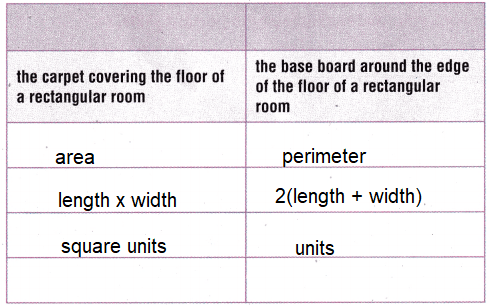Explanation:
The total space or region enclosed inside a rectangle is known as area.
The perimeter of the rectangle is the sum of all its four sides.

Understand Vocabulary

Write the word or term that answers the riddle.

Question 1.
I am the number of unit squares needed to cover a flat surface.
Area
Explanation:
The total space or region enclosed inside a rectangle is known as area.

Question 2.
I am the distance around a figure.
Perimeter
Explanation:
The perimeter of the rectangle is the sum of all its four sides.

Question 3.
I am a unit of area that measures 1 unit by 1 unit.
square unit
Explanation:
A square unit can be defined as the metric unit used to measure area.
Here, for instance, the area of this rectangle is measured in square units using unit squares.

Question 4.
I am a set of symbols that expresses a mathematical rule.
pattern
Explanation:
Pattern is a series or sequence that repeats.

Question 5.
I am an ordered set of numbers or objects.
Term
Explanation:
Terms are the values on which the mathematical operations take place in an expression.
A term can be a constant or a variable or both in an expression.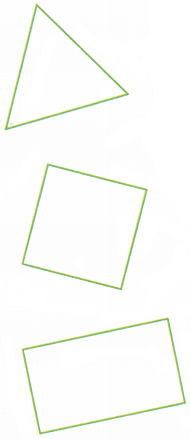Before beginning a lesson on perimeter and area, Lili lists five things she already knows.

Topic: Perimeter and Area

1. Perimeter is the distance around a shape.
2. You can use an inch ruler to measure perimeter.
3. You can use a centimeter ruler to measure perimeter.
4. Area is the number of square units needed to cover a flat surface.
5. You use grid paper to find area.

Writing Think about what you know about perimeter and area. Use grid paper. Draw a rectangle. Find its perimeter. Find its area.

Think
I remember finding the perimeter of a rectangle. I added the lengths of its sides.
50 square units.
Explanation:perimeter of a rectangle  is the added the lengths of its sides of a rectangle
perimeter = AB + BC + CD + DA
= 10 + 5 + 10 + 5 = 30 units
area of a rectangle is the multiplication of sides of a rectangle.
area = AB X BC
= length x width
= 10 x 5 square units

Find the Area
Object of the Game Practice finding the area of different sized rectangles.

Materials

• Inch Rulers
• Rectangle Cards (2 sets)

Set Up

• Each player gets an inch ruler.
• Players shuffle the Rectangle Cards and place them face down in a stack.

Number of Players 2

How to Play

Question 1.
Players take turns drawing a card from the stack until each player has four cards.
Rectangle
Explanation:
A rectangle is a four-sided flat shape where every angle is a right angle (90°).

Question 2.
Players use an inch ruler to measure the length and width of each rectangle to the nearest inch.
Area
Explanation:
The size of a surface is called Area.
The amount of space inside the boundary of a flat (2-dimensional) object such as a triangle or circle,
or surface of a solid (3-dimensional) objects are called Area.

Question 3.
Players find the area for each rectangle and add the areas together.
Square units
Explanation:
A square unit can be defined as the metric unit used to measure area.
Here, for instance, the area of this rectangle is measured in square units using unit square.

Question 4.
The player with the largest sum scores a point.